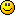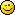#### Topic: Auto Lot Size Calculation

Dear Popov,

Could you please teach me how to add auto lot size calculation (based on percentage) code using metaeditor?

Regards.

do or do not there is no try

#### Re: Auto Lot Size Calculation

Account Percent Entry for MetaTrader 4

1. Rename the first appearance of Entry_Amount input parameter to Entry_Percent. It is at the begging of the expert. Set your starting percent. Change also the parameter comment.
2. Add Maximum_Lots parameter below the Entry_Percent parameter.

Old code:

``static input double Entry_Amount = 0.10; // Entry lots``

New code:

``````static input double Entry_Percent = 2.0; // Entry percent
static input double Maximum_Lots = 0.5; // Maximum entry lots``````

Your code must be something like that:3. Add actual entry amount calculation. Find the "OpenPosition" function and add the following code at its begging.

``````   int margin=(int) MarketInfo(_Symbol,MODE_MARGINREQUIRED);
double Entry_Amount=(Entry_Percent/100)*AccountEquity()/margin;
Entry_Amount=MathMin(Entry_Amount,Maximum_Lots);
Entry_Amount=MathMax(Entry_Amount,0.01);
Entry_Amount=NormalizeDouble(Entry_Amount,2);``````

Your modified function must be something like that:Explanation of the above code:
- line 1 - get the require dmargin.
- line 2 - define and calculate Entry_Amount as percentage of the margin you can invest
- line 3 - assure it is not higher than the  Maximum_Lots;
- line 4 - assure it is not lower than 0.01.
- line 5 - round the number to the second digit.

Now when you run the expert you will see the account percent input parameters:#### Re: Auto Lot Size Calculation

Thank youdo or do not there is no try

#### Re: Auto Lot Size Calculation

Account Percent Entry for MetaTrader 5

The code for MT5 is almost the same as above with only one difference in the OpenPosition function. Instead of AccountEquity() use AccountInfoDouble(ACCOUNT_EQUITY)

Input parameters code:

``````static input double Entry_Percent= 2.0; // Entry percent
static input double Maximum_Lots = 0.5; // Maximum entry lots``````

OpenPosition function code:

``````   int margin=(int)SymbolInfoDouble(_Symbol,SYMBOL_MARGIN_INITIAL);
double Entry_Amount=(Entry_Percent/100)*AccountInfoDouble(ACCOUNT_EQUITY)/margin;
Entry_Amount=MathMin(Entry_Amount,Maximum_Lots);
Entry_Amount=MathMax(Entry_Amount,0.01);
Entry_Amount=NormalizeDouble(Entry_Amount,2);``````

Your code must be something like:#### Re: Auto Lot Size Calculation

Hi Popov, could you please check my EA?

No matter how I change the "entry percent" and "maximum entry lot" value, it always use the "maximum entry lot" size ? so if put 10 on it and 2 on "entry percent", it'll try to open 10 lot, regardless the account balance.

do or do not there is no try

#### Re: Auto Lot Size Calculation

The sample code is corrected.

We find the trading size as percentage from the margin we can invest.

#### Re: Auto Lot Size Calculation

Unfortunately, it is never easy when you deal with MetaTrader.

"SymbolInfoDouble(_Symbol,SYMBOL_MARGIN_INITIAL)" doesn't work and returns 0 for some brokers.

If you have such case, you have to use other means of finding the required margin.

Here is the code I'm using in the FSB Pro experts:

``````   MqlTick tick;
SymbolInfoTick(_Symbol,tick);
double margin=SymbolInfoDouble(_Symbol,SYMBOL_MARGIN_INITIAL);
if(margin<sigma)
if(margin<sigma)

The complete "OpenPosition" function for the MT5 experts will look like that:#### Re: Auto Lot Size Calculation

Thanks a lot, it works and unexpectedly fundo or do not there is no try

#### Re: Auto Lot Size Calculation

Hi Popov, if the broker only allows mini lot (0.1) step/increment, which code should I change?

The backtest show this error : OrderSend error 131, after I searched in Google, it's said the error is due to broker doesn't allow micro lot increment.

I test it using fixed lot version with 0.11 lot and it give the same error, but when I change the lot size to round number such as 0.1 or 0.2, it works well. So I'm sure the problem is the allowed increment

do or do not there is no try

#### Re: Auto Lot Size Calculation

Ah I got it I should change NormalizeDouble(Entry_Amount,2); to NormalizeDouble(Entry_Amount,1);.

But how do I make the EA to detect the minimum allowed increment automatically?

do or do not there is no try

#### Re: Auto Lot Size Calculation

You are correct. You have to make the following changes:

``````    Entry_Amount=MathMax(Entry_Amount,0.1);
Entry_Amount=NormalizeDouble(Entry_Amount,1);``````

This must work. You don't need to set additional increment value because it is most probably 0.1 and when the NormalizeDouble(Entry_Amount,1) function round it to the first decimal digit, it will work fine.

#### Re: Auto Lot Size Calculation

Thanks, but is it posible to make the EA detect minimum alllowed lot size automatically?

do or do not there is no try

#### Re: Auto Lot Size Calculation

Yes, it is possible. You can use the follow function (taken from the FSB Pro scripts):

``````double NormalizeEntrySize(double size)
{
double minlot  = MarketInfo(_Symbol, MODE_MINLOT);
double lotstep = MarketInfo(_Symbol, MODE_LOTSTEP);

if (size <= minlot)
return (minlot);

int steps = (int) MathRound((size - minlot) / lotstep);
size = minlot + steps * lotstep;

if (size >= Maximum_Lots)
size = Maximum_Lots;

size = NormalizeDouble(size, digits);

return (size);
}``````

Put this function somewhere in the file and use it like that:

``Entry_Amount = NormalizeEntrySize(Entry_Amount);``

#### Re: Auto Lot Size Calculation

Hi Popov, I got this error when applying that code, I don't know, may be I applied it incorrectlyThe EA is attached.

do or do not there is no try

#### Re: Auto Lot Size Calculation

This error is because the NormalizeEntrySize is inside the OpenPosition function. MQL doesn't allow nesting functions. The solution is very simple - move NormalizeEntrySize before OpenPosition function.

#### Re: Auto Lot Size Calculation

Thank you so much, it works perfectlydo or do not there is no try

#### Re: Auto Lot Size Calculation

yonkuro

Thanks for asking these features and developing solutions...

Thank you again!

My 'secret' goal is to push EA Studio until I can net 3000 pips per day....

#### Re: Auto Lot Size Calculation

Blaiserboy wrote:

yonkuro

Thanks for asking these features and developing solutions...

Thank you again!

No problem Sir...do or do not there is no try

#### Re: Auto Lot Size Calculation

if i want to have both. Entry amount fix and entry amount with percent. If Lot size percent true...how will be the code for ea studi portfolio or generall normal strats`?

#### Re: Auto Lot Size Calculation

Popov wrote:

Yes, it is possible. You can use the follow function (taken from the FSB Pro scripts):

``````double NormalizeEntrySize(double size)
{
double minlot  = MarketInfo(_Symbol, MODE_MINLOT);
double lotstep = MarketInfo(_Symbol, MODE_LOTSTEP);

if (size <= minlot)
return (minlot);

int steps = (int) MathRound((size - minlot) / lotstep);
size = minlot + steps * lotstep;

if (size >= Maximum_Lots)
size = Maximum_Lots;

size = NormalizeDouble(size, digits);

return (size);
}``````

Put this function somewhere in the file and use it like that:

``Entry_Amount = NormalizeEntrySize(Entry_Amount);``

Is it posible to make the EA detect minimum alllowed lot size and maximum lotsize automatically?
I think the code check the minlot size but what to do if the lotsize reached the maxium allowed from broker?
Is this code correct?

``````double NormalizeEntrySize(double size) {
double minlot  = MarketInfo(_Symbol, MODE_MINLOT);
double maxlot  = MarketInfo(_Symbol, MODE_MAXLOT);
double lotstep = MarketInfo(_Symbol, MODE_LOTSTEP);

if (size <= minlot)
return (minlot);

int steps = (int) MathRound((size - minlot) / lotstep);
size = minlot + steps * lotstep;

if (size >= maxlot || size >=Maximum_Lots)
size = maxlot;

size = NormalizeDouble(size, digits);

return (size);
}   ``````

#### Re: Auto Lot Size Calculation

Dear Popov,

can you please make an update for this old topic? I compared the code from a fresh new strategy with the old one from the EA updated here and it seems to me that the coding is different.

I am very interested (as you know) in using percentages instead of lots and I haven't found a solution yet.

I tried to use FSB because of the percentage, but still I have more other problems there. With Expert Advisor I miss only this function, otherwise it is PERFECT !thanks
Sebastian

#### Re: Auto Lot Size Calculation

i struggling to let run a perfecct function for the entry percent..maybe popov can you make it for the new mt5 porttfolio?